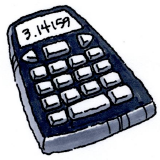### Home > MC1 > Chapter 2 > Lesson 2.1.1 > Problem2-11

2-11.

The test grades for Mrs. Olson's math class are shown below. The sum of all of the test scores is $1663$.

 $1$ $0$ $2$ $3$ $4$ $0$ $5$ $6$ $0\ \ 3\ \ 5$ $7$ $2\ \ 2\ \ 5\ \ 5\ \ 8\ \ 9$ $8$ $0\ \ 0\ \ 1\ \ 5\ \ 5$ $9$ $0\ \ 2\ \ 2\ \ 5\ \ 6\ \ 8$For a reminder on how to read a stem and leaf plot, see the Math Notes box on page 48.
For a reminder on how to calculate the mean, median, and mode, see the Math Notes box on page 56.

Mode is most useful when it shows that one or two test scores occur more frequently than the others.
When there are many modes, they become less important.

1. What is the class average (mean)?

2. What is the median?

3. What is (are) the mode(s)?

4. Is the mode useful for showing central tendency in this case? Explain.